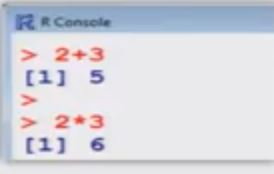# Basics Calculations in R Language

- The assignment operators are the left arrow with dash <- and equal sign =.

> x = 20 assigns the value 20 to x.
Initially only <- was available in R.

> x = 20 assigns the value 20 to x.
> y = x + 2 assigns the value 2 * x to y.
> z = x + y assigns the value x + y to z.

# : The character # marks the beginning of a comment. All characters until the end of the line are ignored.
> # mu is the mean
> # x <- 20 is treated as comment only.

Capital and small letters are different.
> x <- 20 and > x <- 20 are different
The command c (1,2,3,4,5) combines the numbers 1,2,3,4 and 5 to a vector.

R as a calculator> 2 + 3              # Command
 5                  # Output

> 2 * 3              # Command
  6                 # Output

Multiplication and Division x * y , x/y

> c ( 2,3,5,7) * 3
   6 9 15 21

Addition and Subtraction x + y , x - y

> (2,3,5,7) + 10
 12 13 15 17

#### 1 comment:

1.Online learning is an educational mode allowing students to take part in different courses and programs using the internet. In online tuition, students don't need to waste their time going to the lecture halls or classrooms, rather they can decide to learn whatever from any part of the world. For online tuitions you can call - +91-9654271931.

## Categories

Android (23) AngularJS (1) Books (10) C (75) C# (12) C++ (81) Course (1) Downloads (1) Engineering (13) flutter (1) FPL (17) Hadoop (1) HTML&CSS (38) IS (25) Java (89) Leet Code (4) Pandas (1) PHP (20) Projects (17) Python (319) R (69) Software (14) SQL (27)